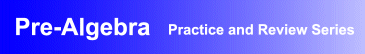Award-Winning

Pre-Algebra Interactive Review and Practice Software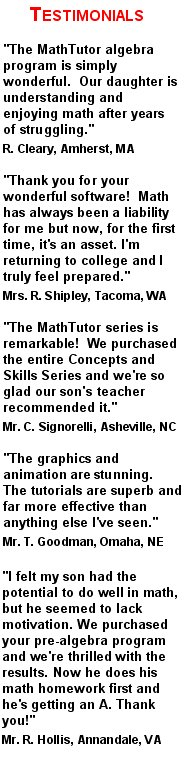DESCRIPTION
PRE-ALGEBRA is the perfect

It is

pre-algebra educational software

at its finest.
Parents seeking a homeschool pre-algebra drill and practice instructional software program will find the program to be perfectly suited to their needs.
Schools seeking

curriculum-based pre-algebra practice and review academic instructional software

will find the program to be ideal.
And others who simply want an interactive pre-algebra practice and drill computer package designed for self study will find the program to be exactly what they were looking for.
PRE-ALGEBRA has been acclaimed for its educational excellence as well as its flexibility. It can be used for self-study and to enhance classroom instruction in high school and middle school math classes.
Designed by math educators and in accordance with math curriculum standards, the program provides interactive pre-algebra drill and practice exercises suitable for students at all levels of ability.
Examples and pre-algebra problem solving exercises progress gradually from the simpler to the more challenging, allowing students to develop outstanding pre-algebra problem solving skills.
• Award-winning, curriculum-based pre-algebra drill and practice software developed by math educators
• Provides dynamic, interactive pre-algebra problem solving exercises that are ideal for self-study, and as a supplement to classroom instruction
• Demonstrated success in boosting students' math skills and comprehension, and proficiency in problem solving
• Network versions contain student record-keeping, grading, and performance analysis features
AVAILABLE FOR WINDOWS
• Windows XP/Vista
• Windows 7/8
• Windows 10
PRICING Single User Version: \$49.95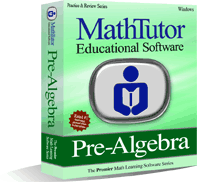TOPICS COVERED
Integers
• The Number Line
• Multiplication Table
• Addition and Subtr. of Pos., Neg. Integers
• Multiplication of Positive Integers
• Word Problems: Multiplication, Division
Fractions
• Least Common Multiple, Greatest Common Divisor
• Equivalent Fractions
• Addition and Subtraction of Fractions
• Addition and Subtraction of Mixed Numbers
• Mult. and Division of Fractions
Decimals
• Addition and Subtraction of Decimals
• Mult. and Division of Decimals
• Equivalent Expressions
• Scientific Notation
Variables
• Words and Symbols
• Words and Equations
• Word Problems: Books
• Statements and Equations
Expressions and Equations
• Substitution
• Satisfying Equations I
• Satisfying Equations II
• Correct Solutions
• Incorrect Equations
• Incorrect Solutions
Algebraic Rules
• Linear Terms I
• Linear Terms II
• Several Variables
• Products
• Simple Powers
• Inequalities
Problem Solving
• Necessary Information
• Unnecessary Information
• Word Problems: TV Sets
• Word Problems: Percent/Ratio
• Word Problems: Formulas
• Units of Measure
• Word Problems: A Garden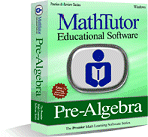Order Now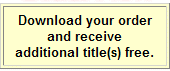TOPICS COVERED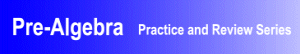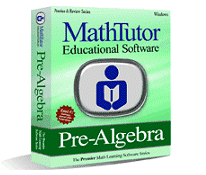TOPICS COVERED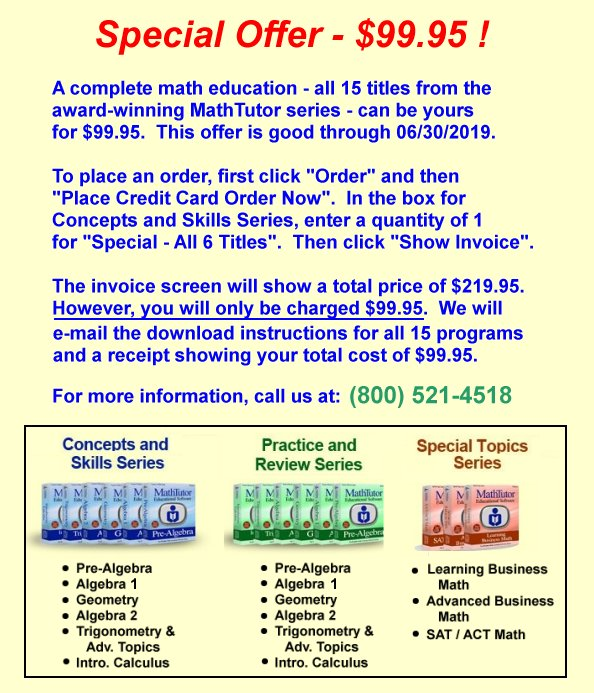×